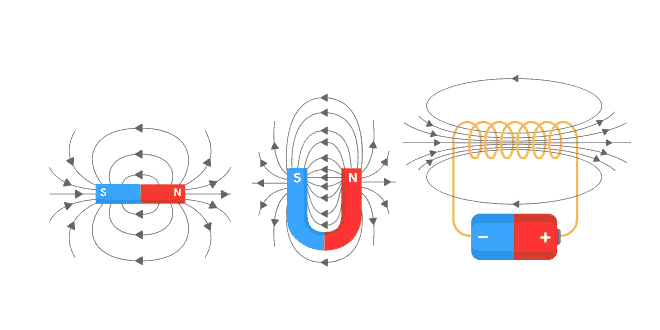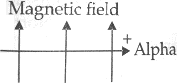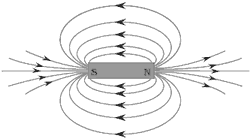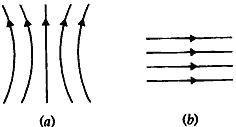Saturday , July 31 2021# Magnetic Effects of Electric Current: 10 Science

#### Question: An alpha particle (+ve charged particle) enters a magnetic field at right angle to it as shown in figure. Explain with the help of a relevant rule, the direction of force acting on the alpha particle.Answer: According to the Fleming’s left hand rule, if the forefinger points in the direction of the magnetic field and the central finger points in the direction of a current, the thumb gives the direction of the force acting on the conductor. So the force will act in the upward direction on the alpha particle.

#### Question: An alternating electric current has a frequency of 50 Hz. How many times does it change its direction in one second? Give reason for your answer.

Answer: 100 times, 50 Hz means 50 cycles per second. One cycle involves two changes of direction. So 50 Hz ac means current changing its direction 100 times in a second.

#### Question: (1). Name the type of electric current generated by the most of the power stations in our country. (2). Why is it preferred over the other type? (3). State the frequency of the power supply generated in India.

1. Alternating current (ac).
2. Alternating current (ac) is preferred because in comparison to direct current (dc), it can be transmitted over long distance effectively.
3. 50 Hz.

#### Question: (1). A compass needle gets deflected when brought near a current carrying conductor. Why? (2). What happens to the deflecting of needle when current in the conductor is increased?

1. Current carrying conductor produces a magnetic field around it.
2. Deflection increases with increases in current.

#### Question: Draw the patterns of magnetic field lines due to a bar magnet. The magnetic field lines are closed curves. Why?Inside the magnet, the direction of field lines is from its south pole to its north pole. So the magnetic field lines are closed curves.

#### Question: (1). In a pattern of magnetic field lines due to a bar magnet, how can the regions of relative strength be identified? (2). Compare the strength of field near the poles and middle of a bar magnet.

1. The relative strength of the magnetic field is shown by the degree of closeness of the field lines.
2. The strength of field near the poles is highest and in the middle of a bar magnet it is lowest.

#### Question: Explain why does a compass needle get deflected when brought near a bar magnet.

Answer: Compass needle is a tiny magnet, so, due to force of repulsion or attraction between the poles of a magnet, there is deflection in the compass needle.

#### Question: Identify the type of magnetic fields represented by the magnetic field lines given below and name the type of conductors which can produce them.1. It represents the magnetic field lines produced by a current carrying circular loop.
2. It represents the magnetic field lines produced by a solenoid.